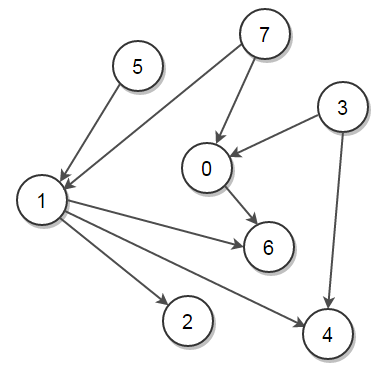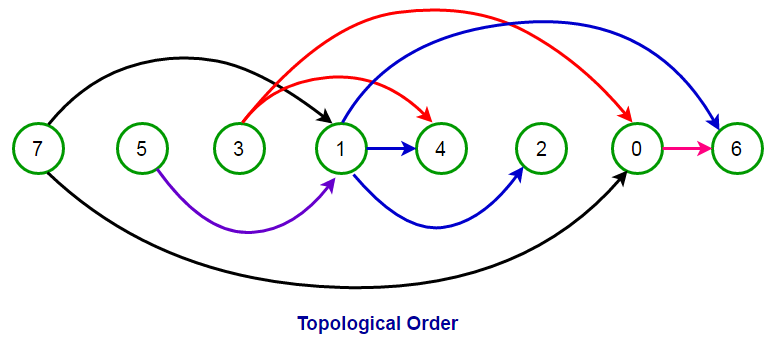# Kahn’s Topological Sort AlgorithmGiven a directed acyclic graph (DAG), print it in Topological order using Kahn’s topological sort algorithm. If the DAG has more than one topological ordering, print any of them. If the graph is not DAG, report that as well.

A Topological sort or Topological ordering of a directed graph is a linear ordering of its vertices such that for every directed edge `uv` from vertex `u` to vertex `v`, `u` comes before `v` in the ordering. Topological order is possible if and only if the graph has no directed cycles, i.e. if the graph is DAG.

For example, consider the following graph:The above graph has many valid topological ordering of vertices like,

7   5   3   1   4   2   0   6
7   5   1   2   3   4   0   6
5   7   3   1   0   2   6   4
3   5   7   0   1   2   6   4
5   7   3   0   1   4   6   2
7   5   1   3   4   0   6   2
5   7   1   2   3   0   6   4
3   7   0   5   1   4   2   6

… and many more

Note that for every directed edge `u —> v`, `u` comes before `v` in the ordering. For example, the pictorial representation of the topological order `[7, 5, 3, 1, 4, 2, 0, 6]` is:Practice this problem

In the previous post, we have seen how to print the topological order of a graph using the Depth–first search (DFS) algorithm. In this post, Kahn’s topological sort algorithm is introduced, which provides an efficient way to print the topological order.

Kahn’s topological sort algorithm works by finding vertices with no incoming edges and removing all outgoing edges from these vertices. Following is a pseudocode for Kahn’s topological sort algorithm taken from Wikipedia:

Kahn’s–Algorithm (graph)

L —> An empty list that will contain the sorted elements
S —> A set of all vertices with no incoming edges (i.e., having indegree 0)

while S is non-empty do
remove a vertex n from S
add n to tail of L
for each vertex m with an edge e from n to m do
remove edge e from the graph
if m has no other incoming edges, then insert m into S
insert m into S

if graph has edges then
return report “graph has at least one cycle”
else
return L “a topologically sorted order”

Note that a DAG has at least one such vertex which has no incoming edges.

How can we remove an edge from the graph or check if a vertex has no other incoming edge in constant time?

The idea is to maintain in-degree information of all graph vertices in a map or an array , say `indegree[]`, for constant-time operations. Here, `indegree[m]` will store the total number of incoming edges to vertex `m`.

• If vertex `m` has no incoming edge and is ready to get processed, its indegree will be 0, i.e., `indegree[m] = 0`.
• To remove an edge from `n` to `m` from the graph, we decrement `indegree[m]` by 1.

Following is the C++, Java, and Python implementation of Kahn’s topological sort algorithm:

Output:

7 5 1 2 3 4 0 6

## Java

Output:

[7, 5, 1, 2, 3, 4, 0, 6]

## Python

Output:

[7, 5, 1, 2, 3, 4, 0, 6]

The time complexity of Kahn’s topological sort algorithm is O(V + E), where `V` and `E` are the total number of vertices and edges in the graph, respectively.

Rate this post

Average rating 4.44/5. Vote count: 68

No votes so far! Be the first to rate this post.

We are sorry that this post was not useful for you!

Tell us how we can improve this post?